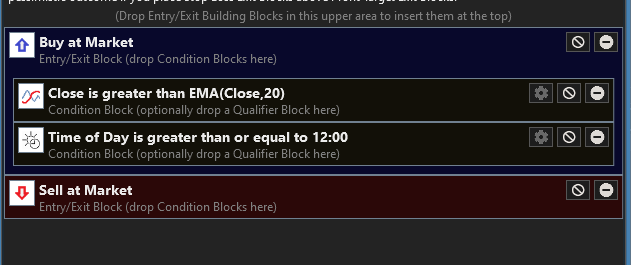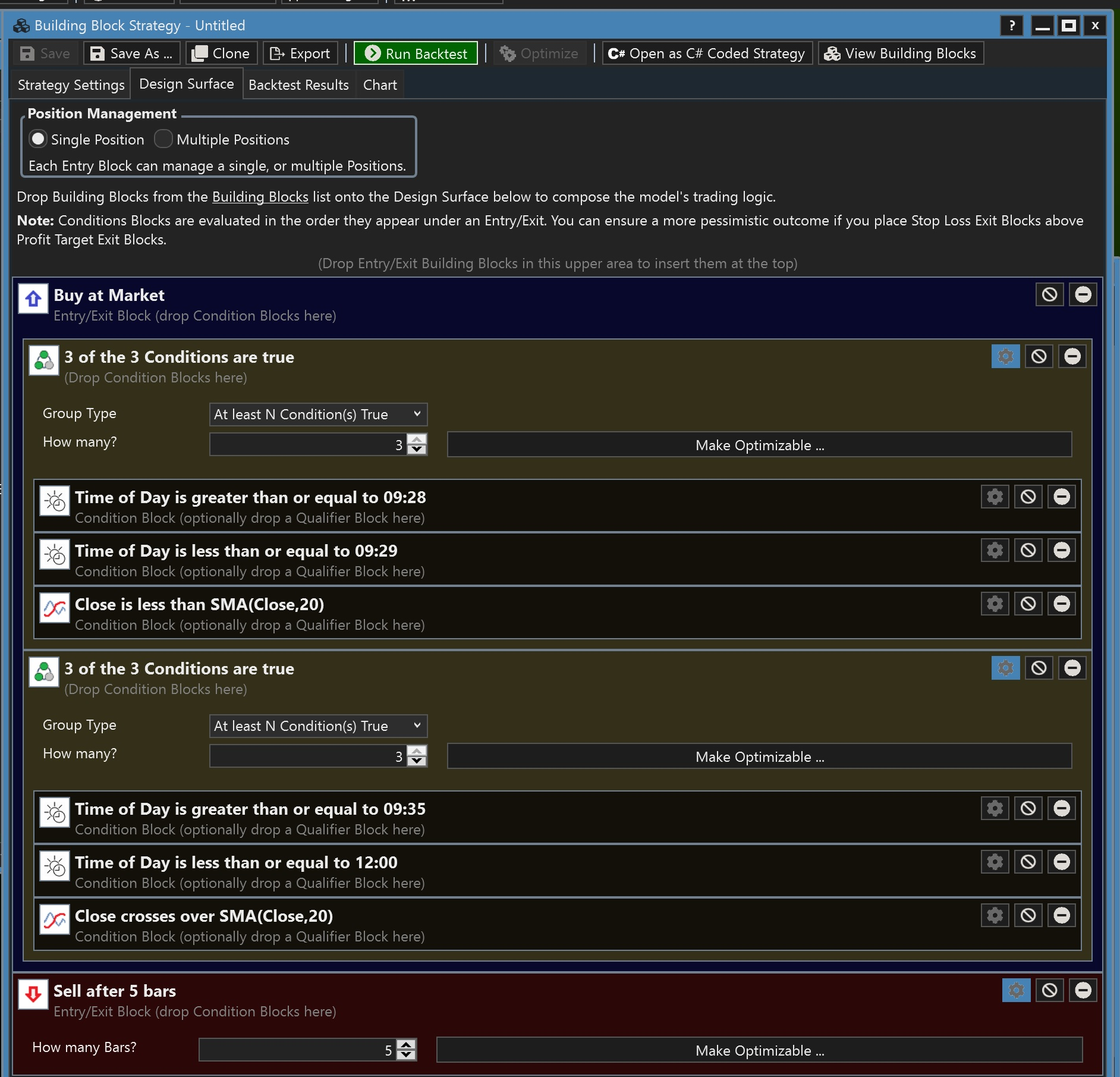Is there a block that will give a Boolean condition? The code will be something like this in C#. (See below) I tried the Time of Day block in PowerPack but it does not let me work it this way.
Of course I can create a C# code snippet and insert it in my strategy, but I was wondering if a block was available. Thanks.

```CODE:if Close > EMA(Indicator) and Time = 9.20AM(Time)
{
PreMarketCond = True
}```
0
220
Solved
13 Replies

Bookmark

Sort
If attach 2 Conditions like this below, they are ANDed together.0
Thanks, I am not getting any trades when I add the Time of Day block.
I do get trades if I have only the Close Grater than EMA block enabled though.
0
Are you running on an intraday scale? Did you enable pre-post market data? Did you open a chart and verify that your selected provider is returning the expected data?
0
In reality what I was looking for is to sets of conditions to occur at different times of day to be true. See code below. Is it possible to do it with two "Multi-Condition" Blocks maybe?

```CODE:        public override void Initialize(BarHistory bars)
{
indicator1 = bars.Close;
indicator2 = new SMA(bars.Close,20);
PlotIndicator(indicator2,new WLColor(0,0,0));

}

public override void Execute(BarHistory bars, int idx)
{
int index = idx;
Position foundPosition0 = FindOpenPosition(0);
bool preMktCondition;
if (foundPosition0 == null)
{
preMktCondition = false;
{
if(bars.DateTimes[index].GetTime() >= 928)
{
if(bars.DateTimes[index].GetTime() <= 929)
{
if (index - 0 >= 0 && indicator1[index] < indicator2[index - 0])
{
preMktCondition = true;
}
}
}
}

MktHrsCondition = false;
{
if(bars.DateTimes[index].GetTime() >= 935)
{
if(bars.DateTimes[index].GetTime() <= 1045)
{
if (index - 0 >= 0 && indicator1[index] < indicator2[index - 0])
{
MktHrsCondition = true;
}
}
}
}

if (preMktCondition) && (MktHrsCondition)
{
}
}```
0
yes, it sounds like that’s what would do it.
0
At the very least you need to promote preMktCondition and MktHrsCondition from the method level to the class level. And, you need to consider resetting both of them prior to 9:28.
0
Thanks, I am trying the concept in Building Blocks. This is what I came up (C# Code below) With Building Blocks will not give me any entries because both Multiple Conditions cancel each other. Any suggestions welcomed. Thank you.```CODE:using WealthLab.Backtest;
using System;
using WealthLab.Core;
using WealthLab.Indicators;
using System.Collections.Generic;

namespace WealthScript2
{
public class MyStrategy : UserStrategyBase
{
public MyStrategy() : base()
{

StartIndex = 20;
}

public override void Initialize(BarHistory bars)
{
indicator1 = bars.Close;
indicator2 = new SMA(bars.Close,20);
PlotIndicator(indicator2,new WLColor(0,0,0));
indicator12 = bars.Close;
indicator22 = new SMA(bars.Close,20);

}

public override void Execute(BarHistory bars, int idx)
{
int index = idx;
Position foundPosition0 = FindOpenPosition(0);
bool condition0;
if (foundPosition0 == null)
{
condition0 = false;
{
count = 0;
if(bars.DateTimes[index].GetTime() >= 928)
{
count++;
}
if(bars.DateTimes[index].GetTime() <= 929)
{
count++;
}
if (index - 0 >= 0 && indicator1[index] < indicator2[index - 0])
{
count++;
}
if (count >= 3)
{
count2 = 0;
if(bars.DateTimes[index].GetTime() >= 935)
{
count2++;
}
if(bars.DateTimes[index].GetTime() <= 1200)
{
count2++;
}
if (indicator12.CrossesOver(indicator22, index))
{
count2++;
}
if (count2 >= 3)
{
condition0 = true;
}
}
}
if (condition0)
{
}
}
else
{
condition0 = false;
{
condition0 = true;
}
if (condition0)
{
Backtester.CancelationCode = 149;
if (idx - foundPosition0.EntryBar + 1 >= 5)
{
ClosePosition(foundPosition0, OrderType.Market, 0,"Sell after 5 bars");
}
}
}

}

public override void NewWFOInterval(BarHistory bars)
{

}

private int count;
private bool mc;
private TimeSeries indicator1;
private IndicatorBase indicator2;
private int count2;
private bool mc2;
private TimeSeries indicator12;
private IndicatorBase indicator22;

private Transaction _transaction;
}
}```
0
First, if you stack Multi-Condition blocks, then they both have to be true, which is not what you want. You need to put each one under its own BuyAtMarket signal. However, both could create a position on the same day.

Recently we discussed the need for an "OR Divider" but I'm not sure if that we turned into a Feature Request or not.

Also, the time condition is VERY restrictive. e.g., If there's no premarket bar at 9:28, then you'll never get a signal.
0
QUOTE:
Recently we discussed the need for an "OR Divider" but I'm not sure if that we turned into a Feature Request or not.

I just turned it into one: Or condition with multiple condition blocks.
0
0
Both multiple conditions have to be true though. It is a reference to a previous event in time. So if previous event = true and current event = true then... Enter.
It might work if I split the premarket session and the regular session into 2 different sessions?
0
I see what you mean - you want an enabling premarket condition that stays true all day AND when the other block is true, then place the trade.

There are some things you'll probably never be able to do with blocks, but maybe we can envision adding a Power Pack "Setup" Qualifier. We'll have to study it!
0
Yes thank you. This will be useful also for near past events of any sort.
0